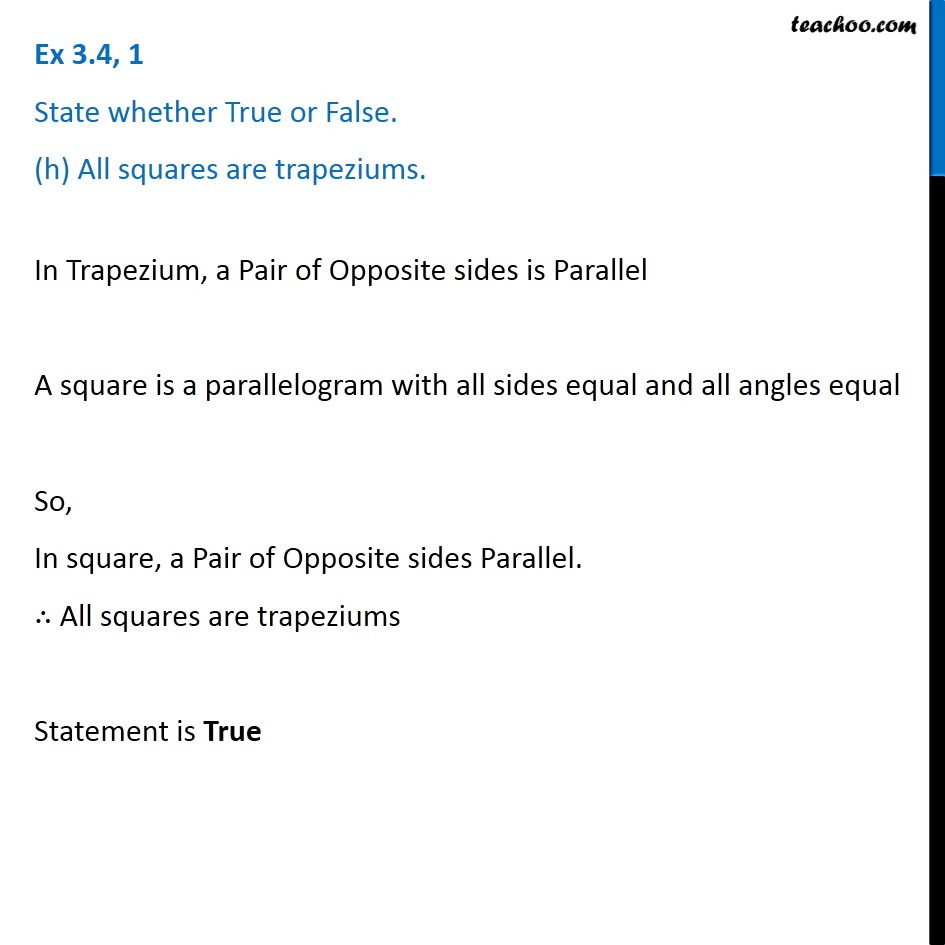1. Chapter 3 Class 8 Understanding Quadrilaterals
2. Serial order wise
3. Ex 3.4

Transcript

Ex 3.4, 1 State whether True or False. (h) All squares are trapeziums. In Trapezium, a Pair of Opposite sides is Parallel A square is a parallelogram with all sides equal and all angles equal So, In square, a Pair of Opposite sides Parallel. ∴ All squares are trapeziums Statement is True